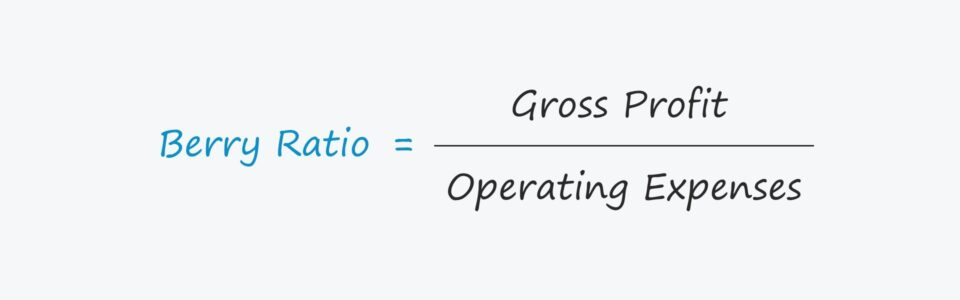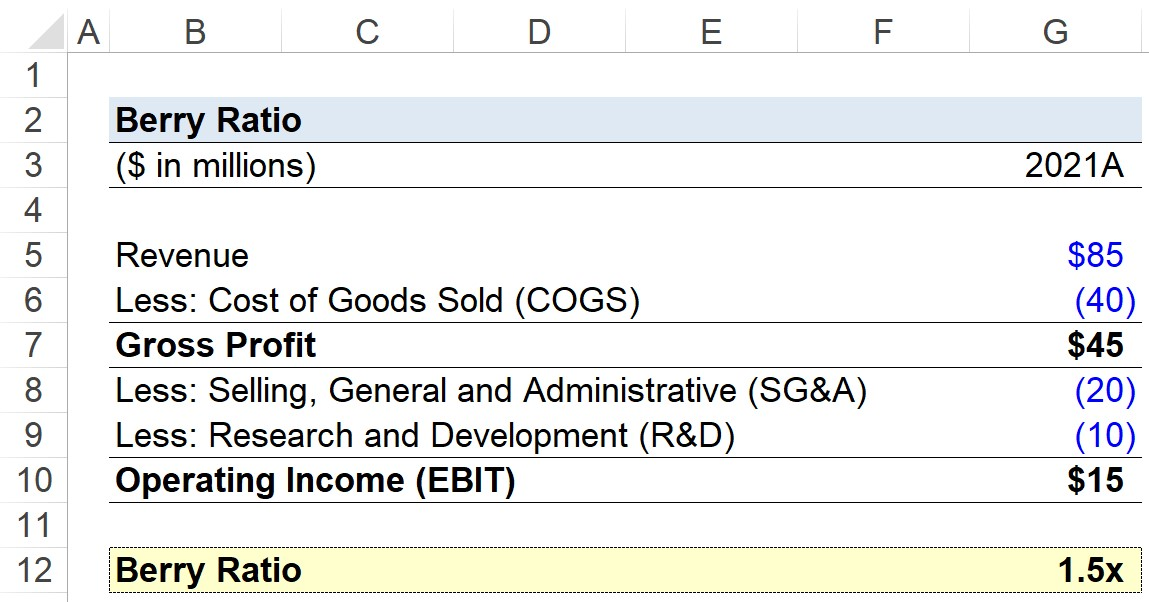# Berry Ratio

Guide to Understanding the Berry Ratio## How to Calculate the Berry Ratio

The Berry ratio is the ratio between a company’s 1) Gross Profit and 2) Operating Expenses.

To calculate the Berry ratio, a company’s gross profit is divided by its total operating expenses.

While the Berry ratio is seldom used in practice, comparing a company’s gross profit to its operating expenses is conceptually tied to various profit measures.

## Berry Ratio Formula

The formula to calculate the Berry Ratio is as follows:

###### Formula
• Berry Ratio = Gross Profit / Operating Expenses

The gross profit is equal to a company’s net revenue minus its cost of goods sold (COGS), which are the incurred costs directly associated with the company’s generation of revenue.

In contrast, operating expenses are the costs incurred as part of the normal course of business, yet are indirectly tied to generating the company’s revenue, e.g. rent and payroll.

## How to Interpret the Berry Ratio

If a company’s Berry ratio is greater than 1.0x, the company is profitable, i.e., generating enough gross profits to offset the operating expenses.

On the other hand, a ratio of less than 1.0x indicates the company is unprofitable and may not be financially stable.

The reason that the metric is not frequently used is that companies with low operating expenses can exhibit misleadingly high ratios, whereas those with higher operating expenses can appear far more financially healthy than in reality.

In fact, the only notable use case of the profitability metric is for purposes related to transfer pricing.

Using the insight derived from the ratio, however, a company can adjust its pricing to ensure sufficient profits are generated to cover not only the operating costs (e.g. COGS and operating expenses) but also non-operating costs such as interest expense.

## Berry Ratio Calculator — Excel Template

We’ll now move to a modeling exercise, which you can access by filling out the form below.Submitting ...

## Berry Ratio Calculation Example

Suppose a company generated \$85 million in revenue for the fiscal year ending 2021.

If the matching direct costs, i.e. the cost of goods sold (COGS), are \$40 million, then the company’s gross profit is \$45 million.

• Revenue = \$85 million
• Cost of Goods Sold (COGS) = \$40 million
• Gross Profit = \$85 million — \$40 million = \$45 million

In terms of the company’s operating expenses, the selling, general and administrative (SG&A) expense was \$20 million while the research and development (R&D) expense is \$10 million.

• SG&A = \$20 million
• R&D = \$10 million
• Total Operating Expense = \$20 million + \$10 million = \$30 million

That said, the company’s operating income — otherwise known as the earnings before interest and taxes (EBIT) — is \$30 million.

• Operating Income (EBIT) = \$45 million — \$20 million — \$10 million = \$15 million

Since the Berry ratio is calculated by dividing the gross profit (\$45 million) by the total operating expense (\$30 million), our hypothetical company’s Berry ratio is 1.5x.

• Berry Ratio = \$45 million / \$30 million = 1.5x

In closing, since the ratio exceeds 1.0x, our model implies that profitability is not an issue for the company. However, the validity of the ratio is entirely dependent on the industry that our company operates within, i.e. whether it is characterized by low or high operating expenses.Step-by-Step Online Course

### Everything You Need To Master Financial Modeling

Enroll in The Premium Package: Learn Financial Statement Modeling, DCF, M&A, LBO and Comps. The same training program used at top investment banks.